# 样本协方差矩阵的特征值的中偏差原理Moderate Deviation Principle of the Eigenvalues of the Sample Covariance Matrix

DOI: 10.12677/AAM.2021.104145, PDF, HTML, XML, 下载: 8  浏览: 32  国家自然科学基金支持

Abstract: The large deviation of eigenvalues of sample covariance matrix has great significance in calculating the bit error probability of Code Division Multiple Access. Throughout the paper, we assume that the i.i.d. real matrix elements of Ckxn satisfy E[Cij]=0, Var(Cij)=1, then the eigenvalue of the sample covariance matrix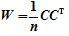is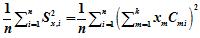, where x is with k coordinates and norm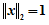. First of all, we prove that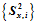satisfies moderate deviations where speed is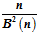and the rate function is, where. Then we use the numerical simulation method to model the theoretical function by python, to verify its reliability. Finally, we use the moderate deviation of sample covariance matrix in the CDMA to compare the advantages and disadvantages of different decoding techniques.

1. 引言

Bai等人     分别研究了元素为独立同分布随机变量时样本协方差矩阵的最大特征值和最小特征值的渐近行为。特别地，当C中的元素服从标准正态分布时，W被称作Wishirt矩阵，Wishart矩阵在统计学中扮演着重要的角色，其样本特征值的大偏差原理可见参考文献 。Guionnet 、Hiai和Petz  研究了速度为 $\frac{1}{{n}^{2}}$ 时Wishart矩阵的样本特征值的大偏差原理。Anne等人   证明了样本协方差矩阵最大最小特征值的大偏差原理，并在  中表明：当 $n\to \infty$，且k固定或者趋于无穷但不快于 $o\left(n/\mathrm{log}\mathrm{log}n\right)$ 时，W的特征值是趋向于1的。由于W的最大最小特征值大偏差恰好就是BEP (Bit Error Probability)，最后Anne等人将样本协方差矩阵的特征值大偏差情况的结果应用到CDMA系统的不同解码方案的建模中，给出了衡量CDMA解码系统优劣的另一种标准，该方法优于之前所使用的SNR (Signal Noise Ratio)，见参考文献 。但样本协方差矩阵的最大最小特征值的大偏差速率函数没有办法直接求解，所以Anne等人在Wishart矩阵中通过中心极限定理逼近的方法得到所求的样本协方差矩阵特征值的速率函数的渐近速率函数，并且在 ${\lambda }_{\mathrm{min}}=0$ 问题没有得到很好的解决，于是本文通过使用中偏差原理的普适性来尝试辅助解决这一问题。

1) 对所有的开集 $G\subseteq X$，有

$\underset{n\to \infty }{\mathrm{lim}}\mathrm{inf}\frac{1}{{a}_{n}}\mathrm{log}ℙ\left({Y}_{n}\in G\right)\ge -\underset{x\in G}{\mathrm{inf}}I\left(x\right),$ (1)

2) 对所有的闭集 $A\subseteq X$，有

$\underset{n\to \infty }{\mathrm{lim}}\mathrm{sup}\frac{1}{{a}_{n}}\mathrm{log}ℙ\left({Y}_{n}\in A\right)\le -\underset{x\in A}{\mathrm{inf}}I\left(x\right),$ (2)

$\left\{{Y}_{n}\right\}$ 在拓扑空间X上服从速度为 $\frac{n}{{B}^{2}\left(n\right)}$ 且速率函数为 $I\left(\cdot \right):X\to {R}^{+}$ 的中偏差原理，其中 $B\left(n\right)$ 是单调递增的数列，并且当 $n\to \infty$ 时满足

$\frac{B\left(n\right)}{n}\to 0,\text{}\frac{B\left(n\right)}{\sqrt{n}}\to \infty ,$ (3)

1) 对所有的开集 $G\subseteq X$，有

$\underset{n\to \infty }{\mathrm{lim}}\mathrm{inf}\frac{n}{{B}^{2}\left(n\right)}\mathrm{log}ℙ\left(\frac{{S}_{n}}{B\left(n\right)}\in G\right)\ge \underset{x\in G}{\mathrm{inf}}I\left( x \right)$

2) 对所有的闭集 $A\subseteq X$，有

$\underset{n\to \infty }{\mathrm{lim}}\mathrm{sup}\frac{n}{{B}^{2}\left(n\right)}\mathrm{log}ℙ\left(\frac{{S}_{n}}{B\left(n\right)}\in A\right)\ge \underset{x\in A}{\mathrm{inf}}I\left( x \right)$

$\exists \delta >0,\text{}E{\text{e}}^{\delta |{X}_{1}|}<+\infty ,$ (4)

$I\left(x\right)=\frac{{x}^{2}}{2\text{Var}\left({X}_{1}\right)}.$ (5)

2. 样本协方差矩阵的特征值的中偏差原理

2.1. 系统模型

$\mathbb{E}\left({C}_{ij}\right)=0,\text{Var}\left({C}_{ij}\right)=1.$ (6)

${\lambda }_{\mathrm{min}}\le 〈x,Wx〉\le {\lambda }_{\mathrm{max}},$

$〈x,Wx〉=\frac{1}{n}{|{C}^{T}x|}^{2}=\frac{1}{n}{\sum }_{i=1}^{n}{\left({\sum }_{m=1}^{k}{x}_{m}{C}_{mi}\right)}^{2}.$

${S}_{x,i}=\underset{m=1}{\overset{k}{\sum }}{x}_{m}{C}_{mi}.$

2.2. 主要结果

$\mathbb{E}\left[{\text{e}}^{ϵ{C}_{11}^{2}}\right]<\infty$ (7)

$\underset{n\to \infty }{\mathrm{lim}}\frac{n}{{B}^{2}\left(n\right)}\mathrm{log}ℙ\left(|\frac{{S}_{n}-n}{B\left(n\right)}|<\epsilon \right)=\frac{{\epsilon }^{2}}{2{\sigma }^{2}}.$ (8)

$\begin{array}{c}ℙ\left(y\right)=\underset{n\to \infty }{\mathrm{lim}}\mathrm{log}{\int }_{ℝ}\mathrm{exp}〈x,y〉/\frac{n}{{B}^{2}\left(n\right)}\text{d}{\mu }_{n}\left(x\right)\\ =\underset{n\to \infty }{\mathrm{lim}}\frac{n}{{B}^{2}\left(n\right)}\mathrm{log}\mathbb{E}\mathrm{exp}\left[\frac{\left({S}_{n}-n\right)y}{B\left(n\right)}\cdot \frac{{B}^{2}\left(n\right)}{n}\right]\\ =\underset{n\to \infty }{\mathrm{lim}}\frac{n}{{B}^{2}\left(n\right)}\mathrm{log}\mathbb{E}\mathrm{exp}{\left[\left({S}_{x,1}^{2}-1\right)\cdot \frac{B\left(n\right)}{n}\right]}^{n}\\ =\underset{n\to \infty }{\mathrm{lim}}\frac{n}{{B}^{2}\left(n\right)}\mathrm{log}\mathbb{E}\mathrm{exp}\left[{S}_{x,1}^{2}\cdot y\cdot \frac{B\left(n\right)}{n}-y\cdot \frac{B\left(n\right)}{n}\right]\\ =\underset{n\to \infty }{\mathrm{lim}}\frac{{n}^{2}}{{B}^{2}\left(n\right)}\left[\Lambda \left(\frac{B\left(n\right)}{n}\cdot y\right)-\frac{B\left(n\right)}{n}\cdot y\right]\end{array}$

$\text{Λ}\left(y\right)=\mathrm{log}\mathbb{E}\mathrm{exp}\left({S}_{x,1}^{2}y\right),$

$f\left(t\right)=\text{Λ}\left(ty\right)=\mathrm{log}\mathbb{E}\mathrm{exp}\left({S}_{x,1}^{2}ty\right)$，计算可以得

${f}^{\prime }\left(t\right)=\frac{\mathbb{E}{S}_{x,1}^{2}y\cdot {\text{e}}^{{S}_{x,1}^{2}ty}}{\mathbb{E}{\text{e}}^{{S}_{x,1}^{2}ty}},$

$t=0$ 可得 ${f}^{\prime }\left(0\right)=y$，再对上式求导可得

${f}^{″}\left(t\right)=\frac{\mathbb{E}{\left({S}_{x,1}^{2}y\right)}^{2}\cdot {\text{e}}^{{S}_{x,1}^{2}ty}-{\left(\mathbb{E}{S}_{x,1}^{2}y\cdot {\text{e}}^{{S}_{x,1}^{2}ty}\right)}^{2}}{{\left(\mathbb{E}\mathrm{exp}{S}_{x,1}^{2}ty\right)}^{2}},$

$ℙ\left(y\right)=\underset{n\to \infty }{\mathrm{lim}}\frac{1}{{\left(\frac{{B}^{2}\left(n\right)}{n}\right)}^{2}}\left[f\left(\frac{B\left(n\right)}{n}\right)-{f}^{\prime }\left(0\right)\frac{B\left(n\right)}{n}\right],$

$ℙ\left(y\right)=\frac{1}{2}{f}^{″}\left(0\right)=\frac{{y}^{2}{\sigma }^{2}}{2},$

$I\left(x\right)=\mathrm{sup}\left(〈x,y〉-\frac{{\sigma }^{2}{y}^{2}}{2}\right)=\frac{{x}^{2}}{2{\sigma }^{2}}.$

$\underset{n\to \infty }{\mathrm{lim}}\frac{n}{{B}^{2}\left(n\right)}\mathrm{log}ℙ\left(|\frac{{S}_{n}-n}{B\left(n\right)}|<\epsilon \right)=\frac{{\epsilon }^{2}}{2{\sigma }^{2}}.$

2.3. 数值模拟

$-\frac{n}{{B}^{2}\left(n\right)}\mathrm{log}ℙ\left(|\frac{{S}_{n}-n}{B\left(n\right)}|<\epsilon \right)\sim \frac{{\epsilon }^{2}}{2{\sigma }^{2}}$

$ℙ\left(|\frac{{S}_{n}-n}{B\left(n\right)}|<\epsilon \right)\sim {\text{e}}^{-\frac{{\left(\epsilon B\left(n\right)\right)}^{2}}{2n{\sigma }^{2}}},$

$P\left(n\right)=ℙ\left(|\frac{{S}_{n}-n}{B\left(n\right)}|<\epsilon \right),$

$Q\left(n\right)={\text{e}}^{-\frac{{\left(\epsilon B\left(n\right)\right)}^{2}}{2n\sigma }}.$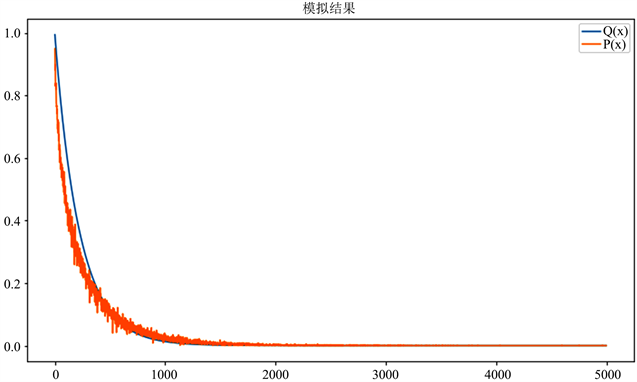Figure 1. Simulation result of Theorem 2.2.1

3. 在CDMA系统中的应用

$s=\underset{m=1}{\overset{k}{\sum }}{r}_{m}+N={C}^{\text{T}}b+N,$

$\stackrel{^}{b}=\frac{1}{n}C{C}^{\text{T}}b+\frac{1}{n}CN.$

$\stackrel{^}{b}=\frac{1}{n}Wb+\frac{1}{n}CN,$

$\stackrel{^}{b}=b+\left(W-I\right)b+\frac{1}{n}CN,$

$b=\underset{j=1}{\overset{k}{\sum }}{\beta }_{j}{w}_{j},$

${\stackrel{^}{b}}^{\left(s\right)}=\underset{\zeta =0}{\overset{s-1}{\sum }}\underset{j=1}{\overset{k}{\sum }}{\left(1-{\lambda }_{j}\right)}^{s}{\lambda }_{j}{\beta }_{j}{w}_{j}=\underset{j=1}{\overset{k}{\sum }}\left(1-{\left(1-{\lambda }_{j}\right)}^{s}\right){\beta }_{j}{w}_{j},$

${{I}^{\prime }}_{SD}\ge \frac{1}{2}-\frac{1}{2}\mathrm{log}2,$

${{I}^{\prime }}_{\text{dec}}\ge \mathrm{log}2,$

${I}_{SD}\ge \mathrm{min}\left\{I\left(\frac{0-n}{{B}_{n}}\right),I\left(\frac{2-n}{{B}_{n}}\right)\right\},$

${I}_{dec}\ge I\left(\frac{0-n}{{B}_{n}}\right),$

$P\left(dec\right)\approx 0.024,$

NOTES

*通讯作者。

  Arcones, M.A. (2003) Moderate Deviations of Empirical Processes. Stochastic Inequalities and Applications, 56, 189-212. https://doi.org/10.1007/978-3-0348-8069-5_13  Bai, Z.D. and Silverstein, J.W. (1998) No Eigenvalues outside the Support of the Limiting Spectral Distribution of Large-Dimensional Sample Covariance Matrices. Annals of Probability, 26, 316-345. https://doi.org/10.1214/aop/1022855421  Bai, Z.D. and Silverstein, J.W. (2004) CLT for Linear Spectral Statistics of Large-Dimensional Sample Covariance Matrices. Annals of Probability, 32, 553-605. https://doi.org/10.1214/aop/1078415845  Bai, Z.D., Silverstein, J.W. and Yin, Y.Q. (1998) A Note on the Largest Eigenvalue of a Large Dimensional Sample Covariance Matrix. Journal of Multivariate Analysis, 26, 166-168. https://doi.org/10.1016/0047-259X(88)90078-4  Bai, Z.D. and Yin, Y.Q. (1993) Limit of the Smallest Eigenvalue of a Large Dimensional Sample Covariance Matrix. Annals of Probability, 21, 1275-1294. https://doi.org/10.1214/aop/1176989118  Fey-den Boer, A., van der Hofstad, R. and Klok, M.J. (2008) Large Deviations for Eigenvalues of Sample Covariance Matrices with Applications to Mobile Communication Systems. Advance in Applied Probability, 40, 1048-1071. https://doi.org/10.1017/S0001867800002962  Guionnet, A. (2002) Large Deviation Asymptotics for Spherical Integrals. Journal of Functional Analysis, 188, 461-515. https://doi.org/10.1006/jfan.2001.3833  Hiai, F. and Petz, D. (1998) Eigenvalue Density of the Wishart Matrix and Large Deviations. Infinite Dimensional Analysis, Quantum Probability, 1, 633-646. https://doi.org/10.1142/S021902579800034X  Fey-den Boer, A., van der Hofstad, R. and Klok, M.J. (2003) Linear Interference Cancellation in CDMA Systems and Large Deviations of the Correlation Matrix Eigenvalues. 10th Symposium on Communications and Vehicular Technology in the Benelux, Eindhoven University of Technology, The Netherlands, 13 November 2003.  Klok, M.J. (2001) Performance Analysis of Advanced Third Generation Receiverss. Ph.D. Thesis, Delft University of Technology, Delft, The Netherlands.  严加安, 彭实戈, 方诗赞, 等. 随机分析选讲[M]. 北京: 科学出版社, 1997.